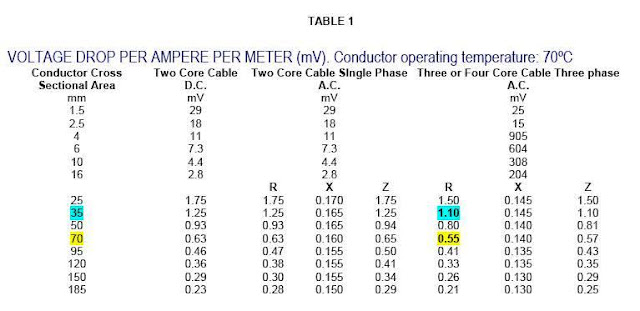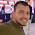# Voltage Drop Calculations- Part One

1- Introduction:

 What is the Voltage Drop? Wires carrying current always have inherent resistance, or impedance, to current flow. Voltage drop is defined as the amount of voltage loss that occurs through all or part of a circuit due to impedance. (see below image)

 Why to calculate the Voltage Drop? Voltage drop becomes important for the following reasons: a- Insure more System efficiency: An applied voltage of 10 percent below rating can result in a decrease in efficiency more than 10 percent For example, fluorescent light output would be reduced by 15 percent, and incandescent light output would be reduced by 30 percent. Induction motors would run hotter and produce less torque. With an applied voltage of 10 percent below rating, the running current would increase 11 percent, and the operating temperature would increase 12 percent. At the same time, torque would be reduced 19 percent. b- Enhance Troubleshooting: If you follow the voltage drop recommendations, you don’t have to guess whether your field measurements indicate a problem or if the voltage is low due to not accommodating voltage drop in the design. c- Enhance Load protection: Under-voltage for inductive loads can cause overheating, inefficiency, and shorter life span. The voltage-drop requirements are needed to ensure the operation of over-current devices to protect conductors and equipment supplied by these systems. d- Enhance equipment capability and reliability: Under-voltage conditions reduce the capability and reliability of motors, lighting sources, heaters, and solid-state equipment.

 What are the factors affecting the voltage drop? There are many factors affecting the voltage drop value as follows: a- The impedance of the conductor: The voltage drop of a circuit is in direct proportion to the impedance of the conductor. If you increase the length of a conductor, you increase its resistance—and you thus increase its voltage drop. Thus long runs often produce voltage drops that exceed NEC recommendations. b- The magnitude of the load current: The voltage drop of a circuit is in direct proportion to The magnitude of the load current. If you increase the load current, you increase the conductor voltage drop. c- Conductor Material: Copper is a better conductor than aluminum and will have less voltage drop than aluminum for a given length and wire size. d- Conductor Size: Larger wire sizes (diameter) will have less voltage drop than smaller wire sizes (diameters) of the same length. e- Conductor Length: Shorter wires will have less voltage drop than longer wires for the same wire size f- The load power factor, g- The type of raceway or cable enclosure, h- The type of circuit (ac, dc, single phase, 3-phase).

2- NEC voltage drop Recommendations

The NEC recommendations concerning Voltage Drop are as follows:

• Section 210.19(A)(1), Informational Note No. 4
• Section 215.2(A)(4), Informational Note No. 2,
• 310.15(A)(1), Informational Note No. 1

And as per NEC 90.5(C), Informational Notes are recommendations, not requirements.

 Rule#1 Contrary to common belief, the NEC generally does not require you to size conductors to accommodate voltage drop. It merely recommends that you adjust for voltage drop when sizing conductors.

 Exception to Rule#1 Unlike electrical distribution systems that supply lighting and appliance branch circuits, the supply systems covered by Article 647 Sensitive Electronic Equipment are subject to mandatory voltage-drop requirements.

 Rule#2 As per NEC Section 210.19(A)(1), Informational Note No. 4 and Section 215.2(A)(4), Informational Note No. 2, Conductors for branch circuits as defined in Article 100, sized to prevent a voltage drop exceeding 3 percent at the farthest outlet of power, heating, and lighting loads, or combinations of such loads, and where the maximum total voltage drop on both feeders and branch circuits to the farthest outlet does not exceed 5 percent, provide reasonable efficiency of operation.(see below image)

 Rule#3 As per NEC Section 310.15 Ampacities for Conductors Rated 0–2000 Volts, Ampacities provided by this section do not take voltage drop into consideration.

The recommended Values for the voltage drop as per NEC code for different applications as in the following table:

 NEC Section Application Voltage Drop Percentage 210.19(A)(1), Informational Note No. 4 and 215.2(A)(4), Informational Note No. 2 Conductors for branch circuits 3% feeders and branch circuits 5% 455.6(A), Informational Note Phase converters 3% 551.73(D) Recreational Vehicles and Recreational Vehicle Parks Total circuit voltage drop = sum of the voltage drops of each serial circuit segment, where the load for each segment is calculated using the load that segment sees and the demand factors of 551.73(A). 647.4(D)(1) Sensitive Electronic Equipment - Fixed Equipment - Conductors for branch circuits 1.5% Sensitive Electronic Equipment -  Fixed Equipment - feeders and branch circuits 2.5% 647.4(D)(2) Sensitive Electronic Equipment - Fixed Equipment - Conductors for branch circuits 1%  and the load connected to the receptacle outlet = 50 % of the branch circuit rating. Sensitive Electronic Equipment -  Fixed Equipment - feeders and branch circuits 2% 695.7(A) Fire Pumps - Starting 15%  (This limitation shall not apply for emergency run mechanical starting) 695.7(B) Fire Pumps - Running 5%  when the motor is operating at 115 percent of the full load current rating of the motor

3- Methods for Voltage Drop Calculations

 Methods for Voltage Drop Calculations There are eight methods for Voltage Drop Calculations as follows:  1- Ohm’s Law Method  2- European method  3- U.S method which divided to:  Circular-mils method, Chapter (9) tables method. 4- Approximate Mathematical method  5- Quick On-Line method  6- Ampere-Feet method  7- Lookup tables  8- Excel spreadsheets

1- Ohm’s Law method

You can determine conductor voltage drop by the Ohm’s for single-phase only from the following Ohm's Law Formula:

VD = I x R

where:

VD = Voltage Drop

I = The load in amperes at 100%, not at 125%, for motors or continuous loads.

R = Conductor Resistance, Chapter 9, Table 8 for dc or Chapter 9, Table 9 for ac.

 Note For conductors 1/0 AWG and smaller, the difference in resistance between dc and ac circuits is so little that it can be ignored. In addition, you can ignore the small difference in resistance between stranded and solid wires.

Example#1:

What is the voltage drop of two 12 AWG THHN conductors that supply a 16A, 120V load located 100 ft from the power supply?

Solution:Ohm's Law Formula is:

VD = I x R

I = 16A

From Chapter 9, Table 9, for 12 AWG THHN conductors, you will find that R = 2 Ω per 1,000 ft,

So, R actual = (2 Ω/1,000 ft) x 200 ft = 0.4 Ω

VD = 16A x 0.4 Ω= 6.4V

2- European method

 Allowable Voltage Drop The nominal maximum acceptable volt drop specified by the IEE Regulations is 2.5% of the system voltage.  Note: the system voltage may be single phase at 215,220,230 or 240 Volts or three phases at 380 or 415 Volts.

 Note This method is used if the cable or conductors meets the european standards.

we have two cases for using this method as follows:

First: For Alternating Current applications

In the European method, the used formula to calculate the voltage drop is:

VD = R x I x L / 1000

Where:

VD: The voltage drop (V),

R: The resistance value from voltage drop per ampere per meter table (mV/A/m) supplied by the cable manufacturer,

I : The load current (A),

L: The length of conductor (m).

The value R is determined from a table provided by the cable manufacturer. (See Table#1 in example#2).

Example#2:

Determine the voltage drop of a 380V, 3 phase circuit with a current of 100A and a length of 150 m and a conductor size of 35 mm.

Solution:

This is a secondary service feed.

The voltage drop of secondary service is limited to 2.5% (380 V x 2.5% = 9.5 Volts).

VD = R x I x L / 1000
Resistance is found on Table 1 below.So, VD = 1.1 x 100 x 150 / 1000 = 16.5 V

The maximum voltage drop allowed is 9.5 V. To determine the size of cable that will be required to meet the voltage drop requirement, determine the value of R that will meet the requirement.
9.5 = R x 100 x 150 / 1000

R = 9.5 x 1000/100 x 150 = 0.633 mV/A/m

Referencing the table provided by the cable manufacturer (Table 1), the cable that has a resistance of 0.633mV/A/m or less is a 70 mm cable with a resistance of 0.55 mV/A/m.

Calculating the voltage drop for the 70mm cable results in:
VD = R x I x L / 1000 = 0.55 x 100 x 150 / 1000 = 8.25 V

Percentage Voltage Drop = 8.25 x 100 / 380 = 2.17 %

Therefore, in order to transmit a 3 phase current of 100A per phase over a length of 150 m, with a total voltage drop equal to or less than the maximum 9.5 volts, a 70 mm cable is needed.

Example#3:

What is the maximum current rating can be applied without exceeding the allowable voltage drop if using 150m of 35mm (Cu.) multi-core PVC cable, 480 V?

Solution:

VD = R x I x L / 1000

Resistance is found on Table 1 below.

VD = 1.1 x I x 150/1000

Allowable VD for 480 V system = 2.5% x 480 = 10.5 V

Therefore, 10.5 = 1.1 x I x 150/1000

I= (10.5 x 1000)/(1.1.x 150) =64 amperes

Second: For Direct Current applications

For cables operating under dc conditions, the appropriate voltage drops may be calculated using the formula:

VD = 2 x R x I x L / 1000

Where:

VD: The voltage drop (V),

R: The resistance value from voltage drop per ampere per meter table (mV/A/m) supplied by the cable manufacturer,

I : The load current (A),

L: The length of conductor (m).

The value R is determined from a table provided by the cable manufacturer. (See Table#1 in example#2).

In the next Article, I will explain Other Methods of Voltage Drop Calculations. Please keep following.

#### 1 comment:

1.جزاك الله خيرا